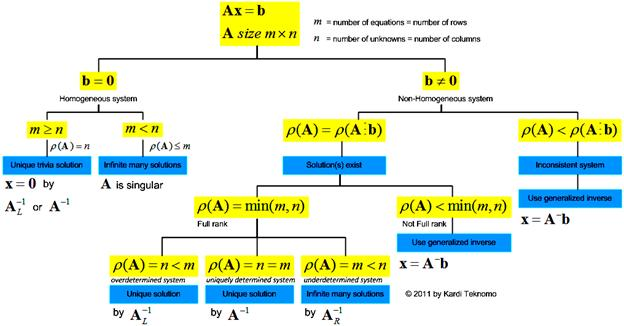## Solving System Linear Equation

Linear equation is an equation in the form of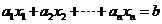The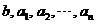are the known constant.
The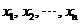are the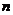unknown variables. The problem in linear equation is to find the values of the unknown variables that satisfy the equation.

For example, when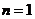,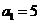and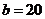we have linear equation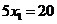and the solution is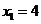.

We may also have several equations and several unknowns that we would like to find out. A linear system is a set of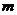linear equations each inunknown. We can write a linear system as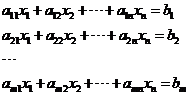When we have many equations and many unknowns, it is easier to represent the linear system into matrix. We put the constant coefficients of the equations into a matrix, and then we can multiply with the unknown to obtain the constants. To transform the system of linear equations into matrix format, you need to reorder the equations according to the order of the unknowns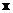and put the coefficients of the unknowns into matrix coefficients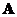. The constants on the right hand side of the equation are written into vector constants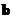. The linear system above can be written as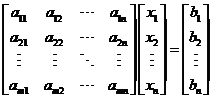Thus, a linear system can be simplified into a matrix product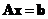A solution of the linear system is an ordered collection ofnumbers that satisfies thelinear equations, which can be written in short as a vector solution.

Example:
Solve a linear system with three equations and three unknowns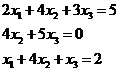The linear system can be written aswhere matrix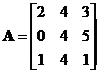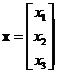and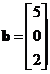The solution of the linear system is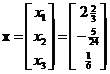The interactive program below will help you to solve a system of linear equations. To use the program, first you need to transform your system of linear equations into matrix format as explained in the example above. Your input is matrix coefficientsand vector constants. Then you click “Solve Linear System Ax=b” button and the program will produce the vector solution. Optionally, you can select your output is either in decimal or in rational format. The rational output is an approximation of the decimal format. When you click “Random Example” button, it will create random input matrix to provide you with more examples of linear system. Note that if the coefficient matrix is singular or nearly singular, you will get only the approximate solution in least square sense using generalized inverse such that the error is minimized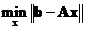.

matrix A                                                         vector b

Report in rational format

## Notes

Some important notes on linear systems are:

• A linear systemis called non-homogeneous system when vectoris not a zero vector. A linear system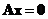is called homogeneous system because vectoris a zero vector.
• Rank of matrixdenoted by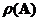is a scalar number to determine whether the linear system is consistent (has a solution), has many solutions or has a unique set of solutions, or inconsistent (has no solution using matrix inverse). Diagram below shows the solution of the system of linear equations based on rank of the coefficient matrixin comparison with the matrix size and rank of the augmented matrix coefficientsand the vector constants,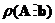.
•has infinitely many non-trivia solutions if and only if the matrix coefficientis singular (i.e. It has no inverse, or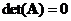), which happens when the number of equations is less than the unknowns (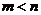). Otherwise, homogeneous system only has unique trivia solution of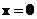. General solution for homogeneous system is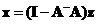where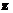is an arbitrary non-zero vector.
• The linear systemis called consistent if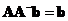. Consistent system can be solved either using matrix inverse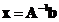, left inverse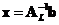or right inverse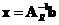. Full rank non-homogeneous system (happen when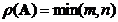) has three possible options:
• When the number of the unknowns in a linear system is the same as the number of equations (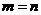), the system is called uniquely determined system. There is only one possible solution to the system computed using matrix inverse.
• When we have more equations than the unknown (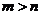), the system is called overdetermined system. The system is usually inconsistent with no possible solution. It is still possible to find unique solution using left inverse.
• When you have more unknowns than the equations (), your system is called underdetermined system. The system usually has infinitely many possible solutions. The standard solution can be computed using right inverse.
• When non-homogeneous systemis not full rank or when the rank of the matrix coefficients is less than the rank of the augmented matrix coefficients and the vector constants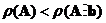then the system is usually inconsistent with no possible solution using matrix inverse. It is still possible to find the approximate least square solution that minimizes the norm of errorusing generalized inverse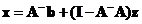whereis an arbitrary non-zero vector.